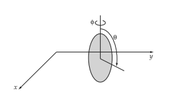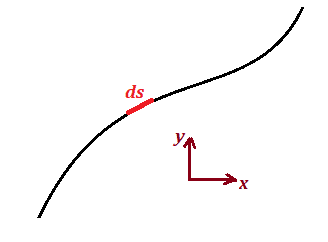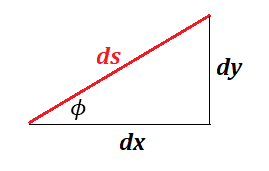# Describing Rolling Constraint for Rolling Disk With No Slipping

• theshape89
In summary, the disk moves along a curve in the x-y plane and its orientation relative to the x-axis is denoted by φ. The distance between the origin and the center of the disk is represented by R. By considering a small element of the curve, ds, the equations (1) and (2) from the first post can be derived, with ds related to the radius of the disk (r) and the amount of rotation (dθ) as it moves along ds. This relationship can be represented by a triangle formed by ds, r, and dθ.

#### theshape89

Homework Statement
A disk rolls without slipping across a horizontal plane. The plane of the disk remains vertical, but it is free to rotate about a vertical axis. What generalized coordinates may be used to describe the motion? Write a differential equation describing the rolling constraint. I've attached a picture below.
Relevant Equations
Let ##\omega## be the angular velocity of a point on the edge of the disk and ##r## the radius of the disk. Then ##v_{tangential}=\omega r##.
Let ##R=\sqrt{x^{2} + y^{2}}##. Then
\begin{align}v_{tangential}&=\frac{dR}{dt} \nonumber\\
&=\frac{dR}{dx}\frac{dy}{dt} + \frac{dR}{dy}\frac{dy}{dt} \nonumber\\
&=\frac{x}{R}\frac{dx}{dt} + \frac{y}{R}\frac{dy}{dt} \nonumber\\
&= cos\phi \frac{dx}{dt} + sin\phi \frac{dy}{dt}.\nonumber \end{align}
Using ##v_{tangential}=\omega r##
##cos\phi \frac{dx}{dt} + sin\phi \frac{dy}{dt} = \frac{d\theta}{dt}r##​
or
##cos\phi dx+ sin\phi dy= d\theta r##​

I'm supposed to end up with
\begin{align}cos\phi dx+ sin\phi dy &= d\theta r\end{align}
and
\begin{align}\frac{dy}{dx}=tan\phi\end{align}.
But I'm not sure how to get (2). If I differentiate (1) w.r.t. ##x## and if the terms with ##\frac{d\phi}{dx}## disappear, I'm still left with ##cos\phi + sin\phi \frac{dy}{dx}=0##, which won't get me to (2).

#### Attachments

•Capture.PNG
1.3 KB · Views: 50
Your working should have partial derivatives in a few places.
Looking at the diagram, it seems self-evident that the angle φ is the current direction of rolling, i.e. tan φ=dy/dx. But I don't understand how in your working you substituted cos φ for x/R and sin φ for y/R. That would imply y/x=tan φ.

haruspex said:
Your working should have partial derivatives in a few places.
Looking at the diagram, it seems self-evident that the angle φ is the current direction of rolling, i.e. tan φ=dy/dx. But I don't understand how in your working you substituted cos φ for x/R and sin φ for y/R. That would imply y/x=tan φ.
Thanks for the reply - looks like I completely messed this one up!

If I start from the start and now say that ##R## is the distance from the origin to the center of the disk, I see what you're saying about ##\frac{dy}{dx}=tan\phi##. Then, expanding ##dR##, I get (remembering to put in the partial derivatives this time!)
$$dR=\frac{\partial R}{\partial x}dx + \frac{\partial R}{\partial y} dy.$$
Where I now get confused is that it seems evident that
$$cos\phi=\frac{\partial x}{\partial R}$$
and
$$sin\phi=\frac{\partial y}{\partial R}.$$
Which then gives me
$$dR=\frac{1}{cos\phi}dx + \frac{1}{sin\phi} dy.$$
Where am I going wrong? I need to be getting
$$dR=cos\phi dx + sin\phi dy.$$

theshape89 said:
it seems evident that
$$cos\phi=\frac{\partial x}{\partial R}$$
No. If we introduce ##\psi## s.t. ##(R,\psi)## is ##(x,y)## in polar coordinates then $$cos\psi=\frac{\partial x}{\partial R}$$ But that is very different from ##\phi##.
Anyway, when using partial derivatives, it helps to bear in mind that strictly speaking one should always identify what variables are being kept constant. Much of the time it is obvious, but when you wrote ##\frac{\partial}{\partial R}##, what was being kept constant?

haruspex said:
No. If we introduce ##\psi## s.t. ##(R,\psi)## is ##(x,y)## in polar coordinates then $$cos\psi=\frac{\partial x}{\partial R}$$ But that is very different from ##\phi##.
Anyway, when using partial derivatives, it helps to bear in mind that strictly speaking one should always identify what variables are being kept constant. Much of the time it is obvious, but when you wrote ##\frac{\partial}{\partial R}##, what was being kept constant?
I believe we are keeping ##\phi## constant, since ##R## is constrained to move along the angle ##\phi##. I think I know what you're getting at; I'm not calculating the differential correctly. I can't in general vary ##x## without varying ##y## since ##R## is constrained to move along ##\phi##. I'll go away and return when I have more...

theshape89 said:
I believe we are keeping ϕ constant
I very much doubt that. It is a rolling wheel, free to change direction.

@theshape89 :

The disk moves along some curve in the x-y plane:Consider a small element ##ds## of the curve. Let ##\phi## be the orientation of ##ds## relative to the x-axis.You should be able to get equations (1) and (2) of your first post directly from this triangle and how ##ds## is related to the radius of the disk ##r##, and the amount ##d\theta## the disk rotates as it travels along ##ds##.